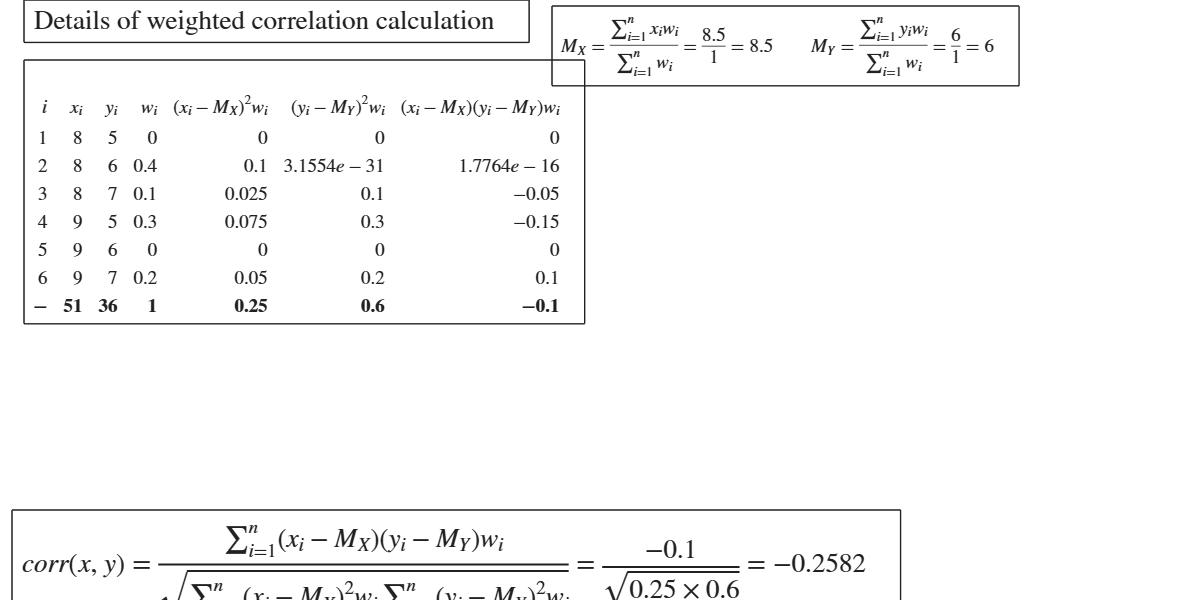# GUIcorr

GUIcorr shows the necessary calculations to obtain the correlation in a GUI.

## Syntax

• out=GUIcorr(x,y)example
• out=GUIcorr(x,y,w)example

## Description

 out =GUIcorr(x, y) Example of unweighted covariance.

 out =GUIcorr(x, y, w) Example 1 of weighted correlation.

## Examples

expand all

### Example of unweighted covariance.

The data below are referred to monthly income of 13 families and their corrisponding free time expenditure (See page 223 of [MRZ]).

% x= monthly income of 13 families.
% y= free time expenditure.
x=[1330 1225 1225 1400 1575 2050 1750 2240 1225 1730 1470 2730 1380];
y=[120 60 30 60 90 150 140 210 30 100 30 270 260];
GUIcorr(x,y)

### Example 1 of weighted correlation.

In this example vectors x y and w are supplied. (See Covariance from Wikipedia)

x=[  8     8     9     9];
y=[6     7     5     7];
w=[0.4000    0.1000    0.3000    0.2000];
y=GUIcorr(x,y,w);

## Related Examples

expand all

###Example 2 of weighted correlation.

In this example first input argument is a table and only this argument is passed. (See Covariance from Wikipedia)

N=[0 0.4 0.1
0.3	 0	0.2];
colnames={'5' '6'	'7'};
rownames={'8','9'};
Ntable=array2table(N,'RowNames',rownames,'VariableNames',colnames);
out=GUIcorr(Ntable);### Another example of weighted correlation.

In this example first input argument is a table and only this argument is passed. (See Correlation from Wikipedia)

N=[0 1/3 0
1/3	 0 1/3];
colnames={'-1' '0' '1'};
rownames={'0','1'};
Ntable=array2table(N,'RowNames',rownames,'VariableNames',colnames);
out=GUIcorr(Ntable);

## Input Arguments

### x — vector of numeric data or table. Vector or table.

Vector containing strictly numerical data. If x is table it contains the contingency table associated with the joint probability distribution. In this case, the second input argument y is not necessary. If x is a table, weighted correation is computed where the weights are the values inside the contingency table.

Data Types: vector of doubles or table

### y — vector of numeric data. Vector.

Vector containing strictly numerical data.

This input argument is not requested if previously input argument x is a table.

Data Types: vector of doubles

### w — weights. Vector.

Vector of the same length of x containing the weights (frequencies) assigned to each observation.

Example: 1:10 

Data Types: vector of doubles

## Output Arguments

### out — description Structure

detailed output to compute the index. struct.

Structure containing the following fields.

Value Description
data

table with n+1 rows (where n is the length of x) containing what is shown in the GUI.

Last row contains the totals.

corr

scalar containing the correlation coefficient.

Milioli, M.A., Riani, M., Zani, S. (2019), "Introduzione all'analisi dei dati statistici (Quarta edizione ampliata)". [MRZ]

Cerioli, A., Milioli, M.A., Riani, M. (2016), "Esercizi di statistica (Quinta edizione)". [CMR]